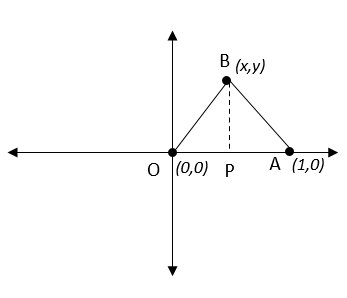# IPMAT Question Paper 2019 | IPM Indore Quants

###### IPMAT Sample Paper | IPMAT Question Paper | Question 35

IPMAT 2019 Question Paper IPM Indore Quantitative Ability. Solve questions from IPMAT 2019 Question Paper from IPM Indore and check the solutions to get adequate practice. The best way to ace IPMAT is by solving IPMAT Question Paper. To solve other IPMAT Sample papers, go here:IPM Sample Paper

Question 35: Two points on a ground are 1 m apart. If a cow moves in the field in such a way that it's distance from the two points is always in ratio 3: 2 then

1. the cow moves in a straight line
2. the cow moves in a circle
3. the cow moves in a parabola
4. the cow moves in a hyperbola

## Best CAT Coaching in Chennai

#### CAT Coaching in Chennai - CAT 2022Limited Seats Available - Register Now!

Let us say that the cow moves between the points O and A which are 1m apart.

For convenience, Let us fix O at (0,0) and A at (1,0).
Let us assume that B is at some point (x,y) where the cow is grazing. Let P be the perpendicular dropped from B on OA.So OP = x and PA = 1-x

OB2= OP2+ BP2= x2+ y2
OB =$$sqrt{x^{2} + y^{2}}\\$ BA2= AP2+ BP2 BA2=$1-x)2+ y2
BA = $$sqrt{$1-x$^{2} + y^{2}}$ Also, $\frac{OB}{BA}\\$ = $\frac{3}{2}\\$ $\frac{\sqrt{x^{2} + y^{2}}}{\sqrt{$1-x$^{2} + y^{2}}}$ = $\frac{3}{2}\\$ $\frac{x^{2} + y^{2}}{$1-x$^{2} + y^{2}}$ = $\frac{9}{4}\\$ 4$x2+ y2) = 9((1−x)2+ y2) = 9 (1 + x2– 2x + y2)

4 x2+ 4 y2= 9 + 9 x2– 18x + 9y2
5x2 + 5y2 – 18x +9 = 0, This is an equation of locus of a Circle.

Hence, the cow moves in a Circular path.

The question is"Two points on a ground are 1 m apart. If a cow moves in the field in such a way that it's distance from the two points is always in ratio 3: 2 then"

##### Hence, the answer is the cow moves in a circle

Choice B is the correct answer

##### Where is 2IIM located?

2IIM Online CAT Coaching
A Fermat Education Initiative,
58/16, Indira Gandhi Street,
Kaveri Rangan Nagar, Saligramam, Chennai 600 093

##### How to reach 2IIM?

Mobile: (91) 99626 48484 / 94459 38484
WhatsApp: WhatsApp Now
Email: info@2iim.com